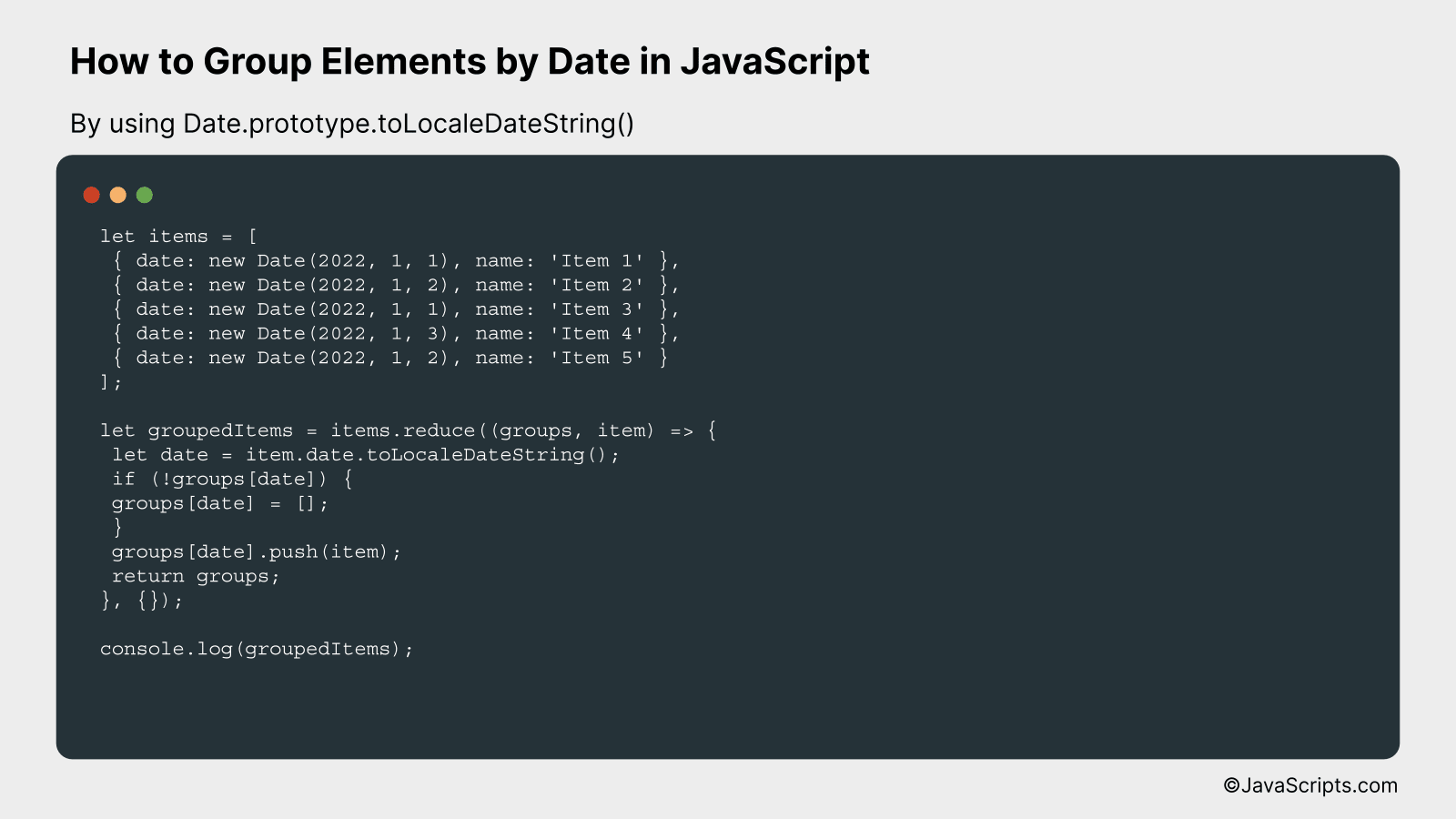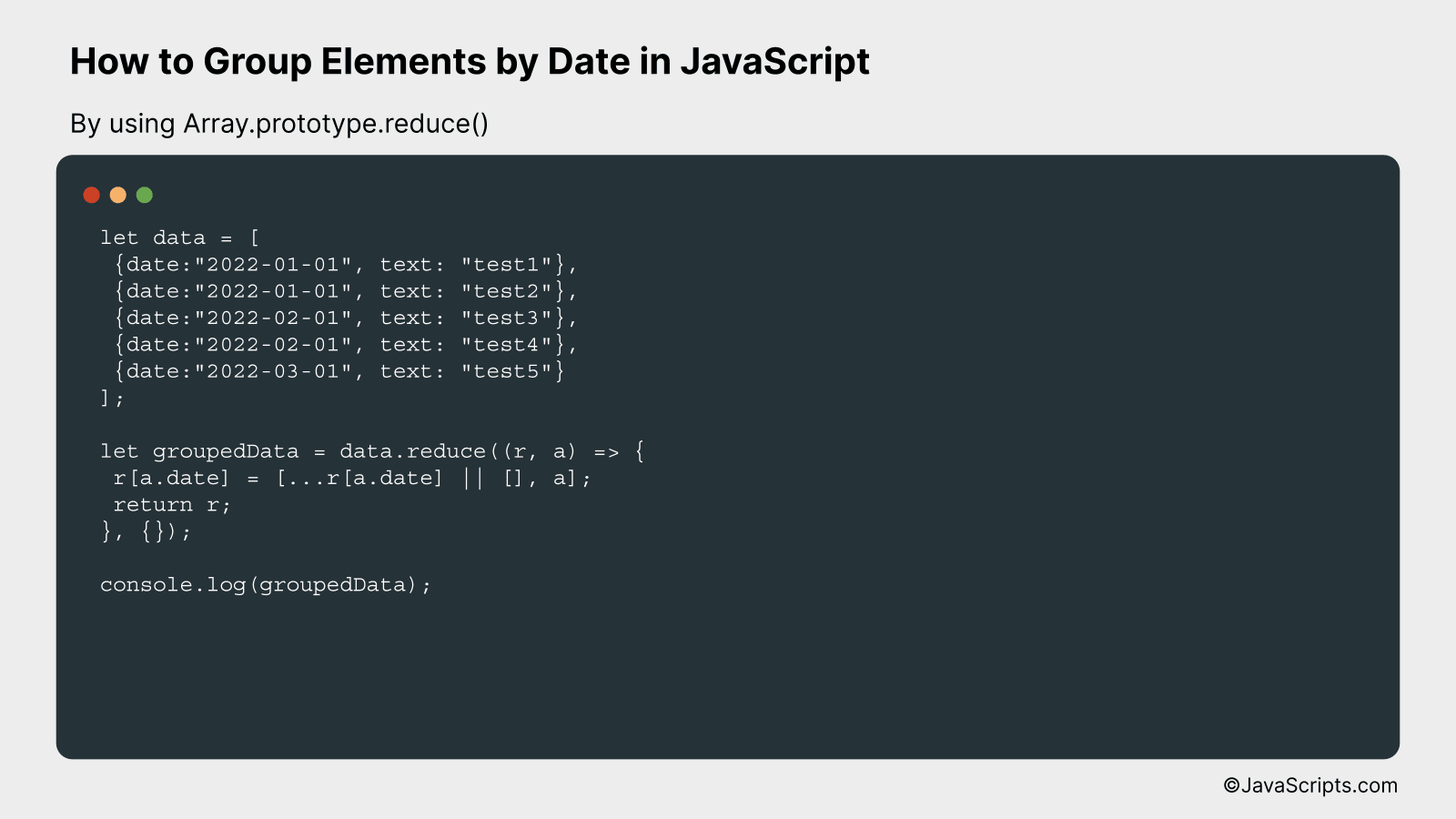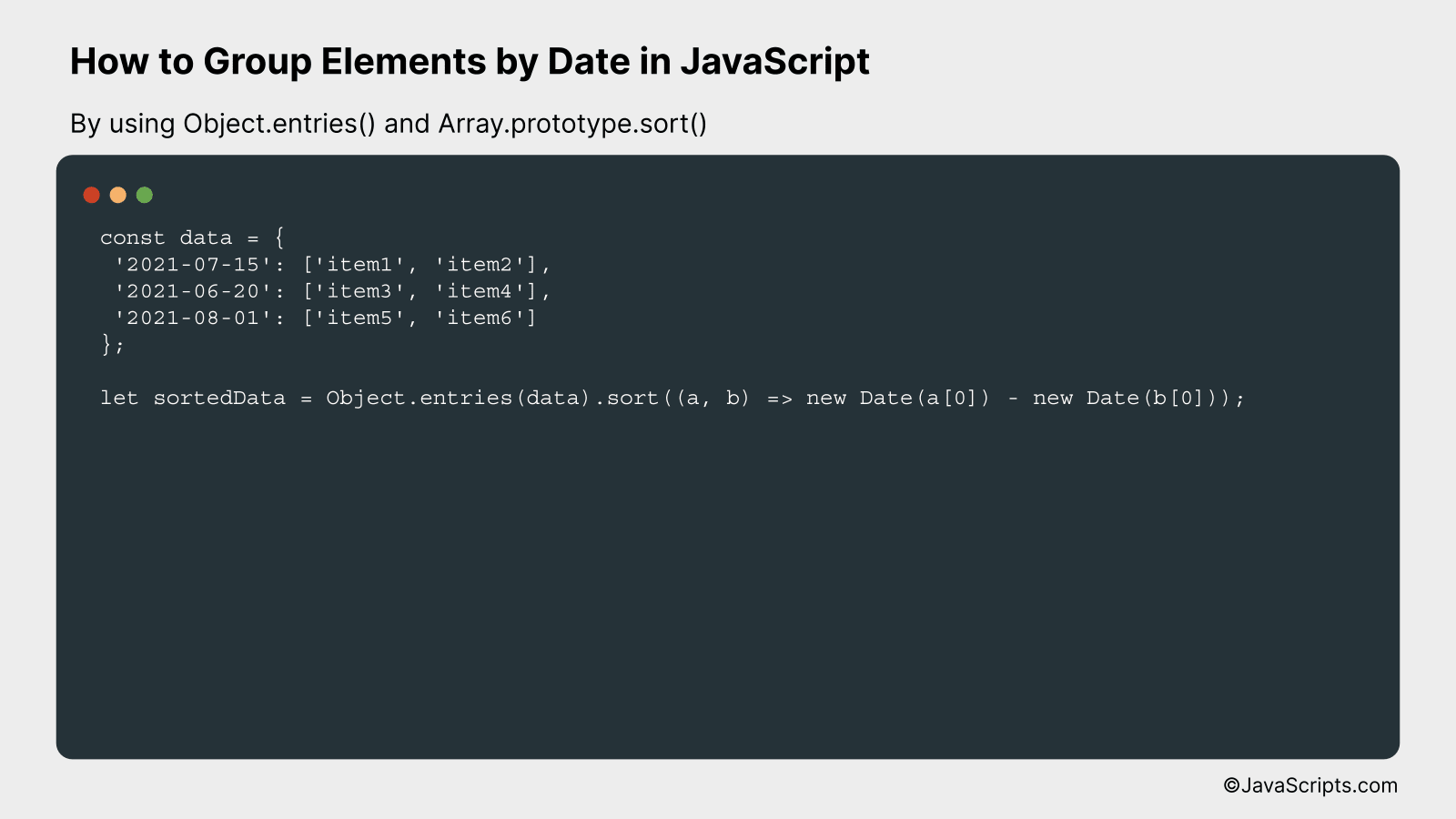# How to Group Elements by Date in JavaScript

Managing data is a common challenge in programming, especially when dealing with dynamic information like dates. It’s crucial to find effective ways to organize this data for better usability and accessibility.

Let’s dive into how you can group elements by date using JavaScript. We’ll take a journey together, exploring simple and practical methods for organizing your date-based data. Don’t worry if you’re a beginner, we’re keeping it super straightforward and easy to understand.

In this post, we will be looking at the following 3 ways to group elements by date in JavaScript:

• By using Date.prototype.toLocaleDateString()
• By using Array.prototype.reduce()
• By using Object.entries() and Array.prototype.sort()

Let’s explore each…

## #1 – By using Date.prototype.toLocaleDateString()We will group elements by their date in JavaScript by converting each date to a unique string using Date.prototype.toLocaleDateString() method, then using this string as a key in an object to group associated elements together. For a better understanding, let’s see this in an example:

``````
let items = [
{ date: new Date(2022, 1, 1), name: 'Item 1' },
{ date: new Date(2022, 1, 2), name: 'Item 2' },
{ date: new Date(2022, 1, 1), name: 'Item 3' },
{ date: new Date(2022, 1, 3), name: 'Item 4' },
{ date: new Date(2022, 1, 2), name: 'Item 5' }
];

let groupedItems = items.reduce((groups, item) => {
let date = item.date.toLocaleDateString();
if (!groups[date]) {
groups[date] = [];
}
groups[date].push(item);
return groups;
}, {});

console.log(groupedItems);
``````

### How it works

This code works by using the array reduce method to iterate over the list of items and group them by their date. Each date is converted to a string using the Date.prototype.toLocaleDateString() method which serves as a unique key in the group’s object.

• First, we define an array of items, each having a ‘date’ and ‘name’ property.
• We then call the reduce method on the ‘items’ array. The reduce function takes in two parameters – an accumulator (which is an object that will hold our groups) and the current item in the iteration.
• For each item, we convert its date to a string using ‘toLocaleDateString()’ method. This string will be used as a key in our groups object.
• We then check if this key already exists in our groups object. If it doesn’t, we initialize it with an empty array.
• We then push the current item into the array associated with the current date key in the groups object.
• Finally, we return the groups object from the reduce function. This object will hold our items grouped by date.

## #2 – By using Array.prototype.reduce()We will use the Array.prototype.reduce() function in JavaScript, which processes an array’s elements by accumulating them into a single output value. In our case, we will accumulate elements into groups by their dates. For example, if we have an array of objects, each having a ‘date’ and ‘text’ property, we will group these objects by their ‘date’ values.

``````
let data = [
{date:"2022-01-01", text: "test1"},
{date:"2022-01-01", text: "test2"},
{date:"2022-02-01", text: "test3"},
{date:"2022-02-01", text: "test4"},
{date:"2022-03-01", text: "test5"}
];

let groupedData = data.reduce((r, a) => {
r[a.date] = [...r[a.date] || [], a];
return r;
}, {});

console.log(groupedData);
```
```

### How it works

The Array.prototype.reduce() function in JavaScript takes a callback function as its first argument. This callback is provided with two parameters: the accumulator (in our case, ‘r’) and the current value (in our case, ‘a’). The accumulator is the value that we are building up during the reduction process. The current value is the current element being processed in the array.

• First, an empty object {} is passed as the initial value of the accumulator ‘r’.
• During each iteration, the date of the current item ‘a.date’ is being used as a key in the accumulator object.
• If this key already exists in the accumulator, we spread its current contents and append the current item ‘a’ to it. If the key does not exist, a new array containing only the current item ‘a’ is created.
• Finally, the updated object ‘r’ is returned at the end of each iteration. This updated object becomes the accumulator for the next iteration.
• When all items in the array have been processed, the reduce function returns the final value of the accumulator, which is an object that groups the original items by their dates.

## #3 – By using Object.entries() and Array.prototype.sort()This approach will convert the original object into an array of key-value pairs using Object.entries(), and then sort this array based on dates using Array.prototype.sort(). For better understanding, let’s consider an example where we have an object with dates as keys and some data as values.

``````
const data = {
'2021-07-15': ['item1', 'item2'],
'2021-06-20': ['item3', 'item4'],
'2021-08-01': ['item5', 'item6']
};

let sortedData = Object.entries(data).sort((a, b) => new Date(a) - new Date(b));
```
```

### How it works

The above code takes an object where the keys are dates and values are data associated with those dates. It converts the object to an array of key-value pairs and then sorts the array based on the dates.

• First, `Object.entries(data)` is used to transform the object into an array. Each item in the array is a key-value pair from the original object. In this case, the keys are dates and values are arrays of items.
• Next, `Array.prototype.sort()` is used to sort the array. In the sorting function, `(a, b) => new Date(a) - new Date(b)`, `a` and `b` represent the keys (i.e., dates) of the two elements being compared. `new Date(a) - new Date(b)` is used to convert these keys to date objects and then find the difference. If the difference is negative, `a` is sorted to an index lower than `b`, i.e., `a` comes first. If it’s positive, `a` is sorted to an index higher than `b`, i.e., `b` comes first. If the difference is 0, their position is left unchanged.
• The result, `sortedData`, is the sorted array of key-value pairs.

Related:

In closing, managing and grouping elements by date in JavaScript isn’t as complex as it seems initially. By leveraging inbuilt JavaScript methods and writing a few custom functions, we can readily create date-sorted groups.

Remember, practice is key in mastering these concepts. Keep exploring different methods and scenarios to further enhance your skills. Happy coding!